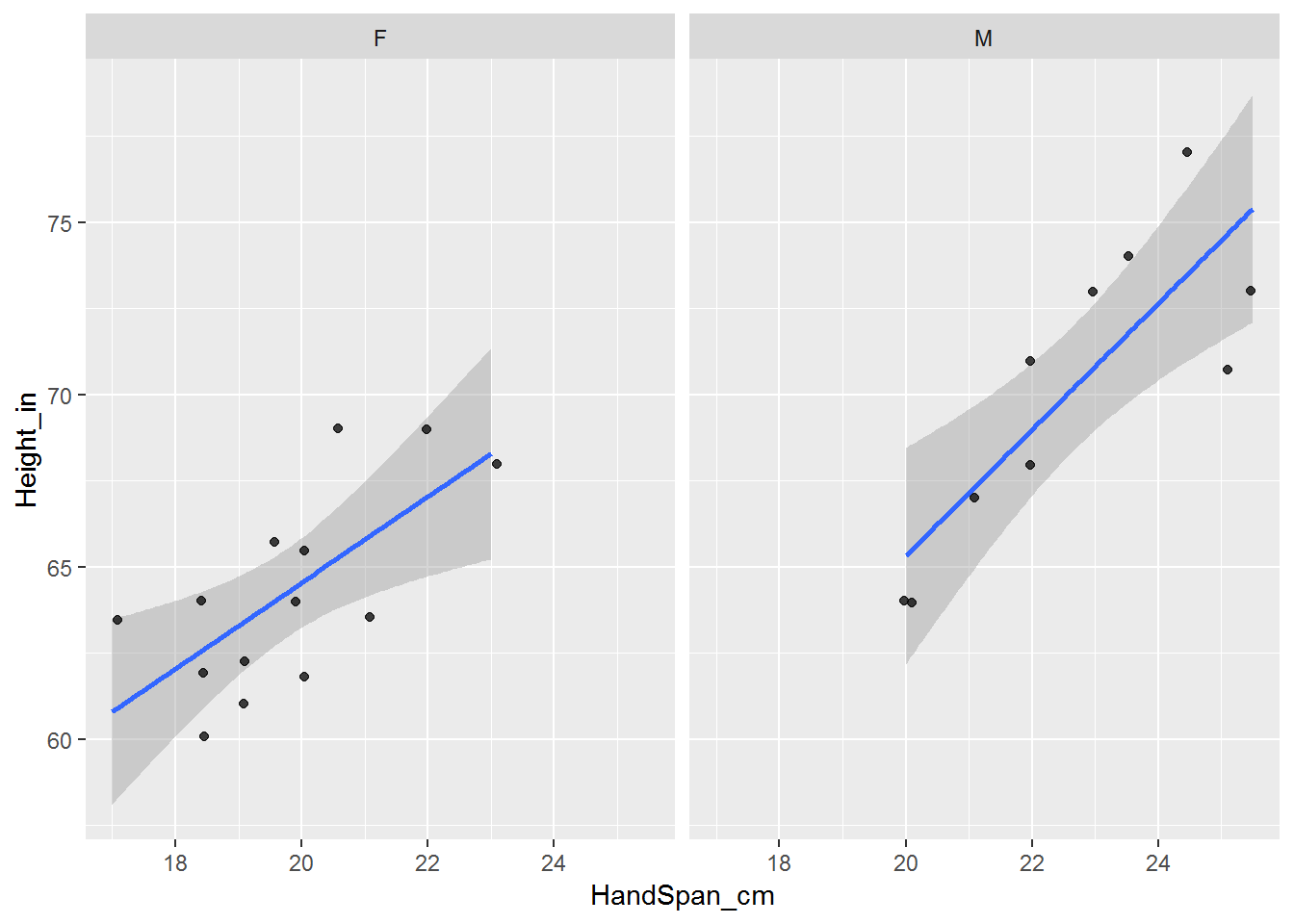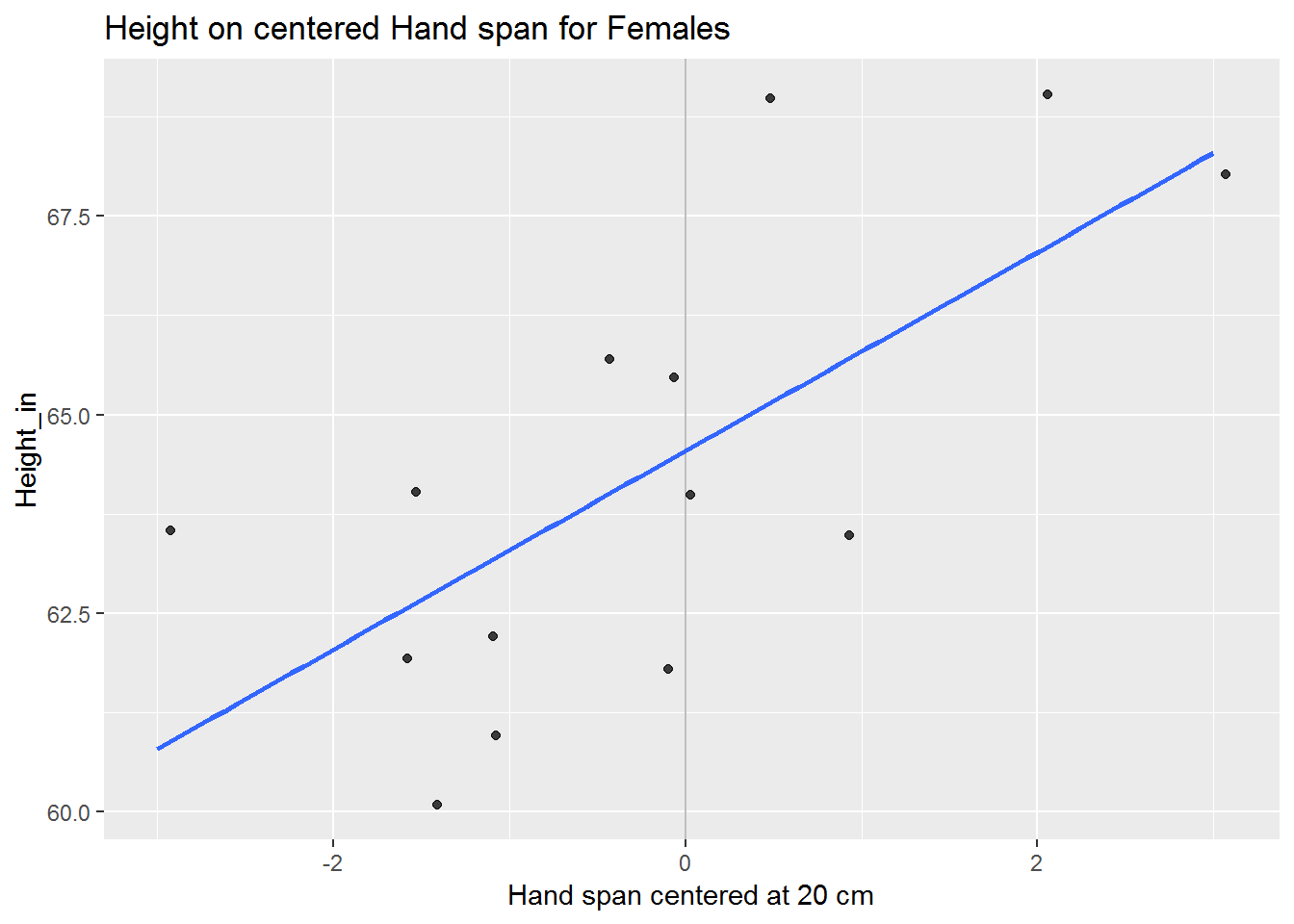This assignment is separate from your project. Include your answers in this document in the sections below the rubric.

Plan: Use the height and hand span data collected in class to fit and interpret a simple linear regression model: plot the data, center the explanatory variable HandSpan_cm, fit a simple linear regression model, and interpret the parameter estimate table.

Rubric

Answer the questions with the data example that we collected earlier in the semester.

Read data and plot by gender

library(tidyverse)
## -- Attaching packages --------------- tidyverse 1.2.1 --
## v ggplot2 3.1.0       v purrr   0.3.2
## v tibble  2.1.1       v dplyr   0.8.0.1
## v tidyr   0.8.3       v stringr 1.4.0
## v readr   1.3.1       v forcats 0.4.0
## -- Conflicts ------------------ tidyverse_conflicts() --
## x dplyr::filter() masks stats::filter()
## x dplyr::lag()    masks stats::lag()
dat.hand <-
## Parsed with column specification:
## cols(
##   Table = col_double(),
##   Person = col_double(),
##   Gender_M_F = col_character(),
##   Height_in = col_double(),
##   HandSpan_cm = col_double()
## )
dat.hand <- na.omit(dat.hand)
dat.hand$Gender_M_F <- factor(dat.hand$Gender_M_F, levels = c("F", "M"))

str(dat.hand)
## Classes 'tbl_df', 'tbl' and 'data.frame':    24 obs. of  5 variables:
##  $Table : num 1 1 1 1 1 1 2 2 2 2 ... ##$ Person     : num  1 2 3 4 5 6 1 2 3 4 ...
##  $Gender_M_F : Factor w/ 2 levels "F","M": 1 2 1 1 1 1 1 1 2 1 ... ##$ Height_in  : num  64 73 61.9 63.5 60.1 61 69 69 71 65.5 ...
##  \$ HandSpan_cm: num  18.5 25.5 18.5 17 18.5 19 22 20.5 22 20 ...
##  - attr(*, "na.action")= 'omit' Named int  7 8 9 18 26 27 31 32 33 34 ...
##   ..- attr(*, "names")= chr  "7" "8" "9" "18" ...

Plot data for Height_in vs HandSpan_cm for Females and Males.

library(ggplot2)
p <- ggplot(dat.hand, aes(x = HandSpan_cm, y = Height_in))
# linear regression fit and confidence bands
p <- p + geom_smooth(method = lm, se = TRUE)
# jitter a little to uncover duplicate points
p <- p + geom_jitter(position = position_jitter(.1), alpha = 0.75)
# separate for Females and Males
p <- p + facet_wrap(~ Gender_M_F, nrow = 1)
print(p)Change the code to use Males for the remaining analysis.

# choose one by uncommenting the one you want to use and commenting the other:
dat.use <-
dat.hand %>%
filter(Gender_M_F == "F")    # use Females
#filter(Gender_M_F == "M")    # use Males

Center the explanatory variable HandSpan_cm

Recentering the $$x$$-variable doesn’t change the model, but it does provide an interpretation for the intercept of the model. For example, if you interpret the intercept for the regression lines above, it’s the “expected height for a person with a hand span of zero”, but that’s not meaningful.

(2 p) Choose a sensible value to center your data on.

A good choice is a nice round number near the mean (or center) of your data. This becomes the value for the interpretation of your model intercept (value of $$y$$ when $$x=0$$).

I use the value 20, which means that our new HandSpan_cm_centered is 0 for a Female with a “typical” handspan of 20 cm, -2 for 18 cm, and +2 for 22 cm.

dat.use <-
dat.use %>%
mutate(
HandSpan_cm_centered = HandSpan_cm - 20
)

# let's look at the data to see that the centered variable makes sense
dat.use
## # A tibble: 14 x 6
##    Table Person Gender_M_F Height_in HandSpan_cm HandSpan_cm_centered
##    <dbl>  <dbl> <fct>          <dbl>       <dbl>                <dbl>
##  1     1      1 F               64          18.5                 -1.5
##  2     1      3 F               61.9        18.5                 -1.5
##  3     1      4 F               63.5        17                   -3
##  4     1      5 F               60.1        18.5                 -1.5
##  5     1      6 F               61          19                   -1
##  6     2      1 F               69          22                    2
##  7     2      2 F               69          20.5                  0.5
##  8     2      4 F               65.5        20                    0
##  9     2      7 F               61.8        20                    0
## 10     2      8 F               68          23                    3
## 11     3      1 F               62.2        19                   -1
## 12     3      3 F               64          20                    0
## 13     3      6 F               65.7        19.5                 -0.5
## 14     3      7 F               63.5        21                    1

Fit a simple linear regression model

# fit model
lm.fit <- lm(Height_in ~ HandSpan_cm_centered, data = dat.use)

Here’s the data you’re using for the linear regression, with the regression line and confidence and prediction intervals.

library(ggplot2)
p <- ggplot(dat.use, aes(x = HandSpan_cm_centered, y = Height_in))
p <- p + geom_vline(xintercept = 0, alpha = 0.25)
# linear regression fit and confidence bands
p <- p + geom_smooth(method = lm, se = FALSE)
# jitter a little to uncover duplicate points
p <- p + geom_jitter(position = position_jitter(.1), alpha = 0.75)
p <- p + labs(
title = "Height on centered Hand span for Females"
, x = "Hand span centered at 20 cm"
)
print(p)Interpret the parameter estimate table

Here’s the parameter estimate table.

We’re estimating the $$\beta$$ parameter coefficients in the regression model $$\hat{y}_i = \beta_0 + \beta_1 x_i$$.

summary(lm.fit)
##
## Call:
## lm(formula = Height_in ~ HandSpan_cm_centered, data = dat.use)
##
## Residuals:
##     Min      1Q  Median      3Q     Max
## -2.7446 -1.9820 -0.4193  1.6678  3.8305
##
## Coefficients:
##                      Estimate Std. Error t value Pr(>|t|)
## (Intercept)           64.5446     0.5978 107.978  < 2e-16 ***
## HandSpan_cm_centered   1.2498     0.3938   3.173  0.00802 **
## ---
## Signif. codes:  0 '***' 0.001 '**' 0.01 '*' 0.05 '.' 0.1 ' ' 1
##
## Residual standard error: 2.206 on 12 degrees of freedom
## Multiple R-squared:  0.4563, Adjusted R-squared:  0.411
## F-statistic: 10.07 on 1 and 12 DF,  p-value: 0.00802

(2 p) Assuming the model fits well, complete this equation (fill in the $$\hat{\beta}$$ values below with values from the table) with the appropriate numbers from the table above (3 numbers: each beta and the HandSpan centering value).

The regression line is $$\hat{\textrm{Height_in}} = \hat{\beta}_0 + \hat{\beta}_1 \textrm{(HandSpan_cm - 20)}$$.

(2 p) State the hypothesis test related to the slope of the line, indicate the p-value for the test, and state the conclusion.

Words and notation:

• Words:

• Notation: $$H_0:\beta_? = ?$$ vs $$H_A:\beta_? \ne ?$$

(2 p) Interpret the slope coefficient in the context of the model by changing this generic sentence to relate to your hypothesis.

For each unit increase in $$x$$, we expect an increase of beta1 in $$y$$.

(2 p) State and interpret the $$R^2$$ value.